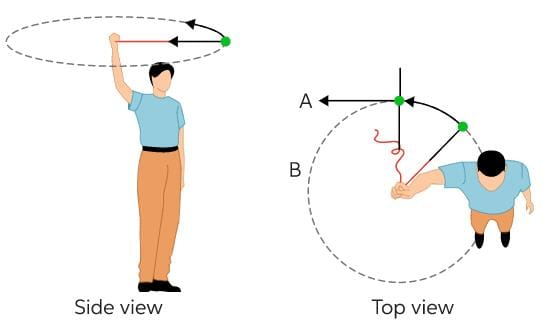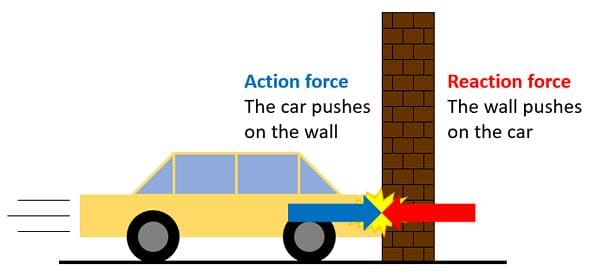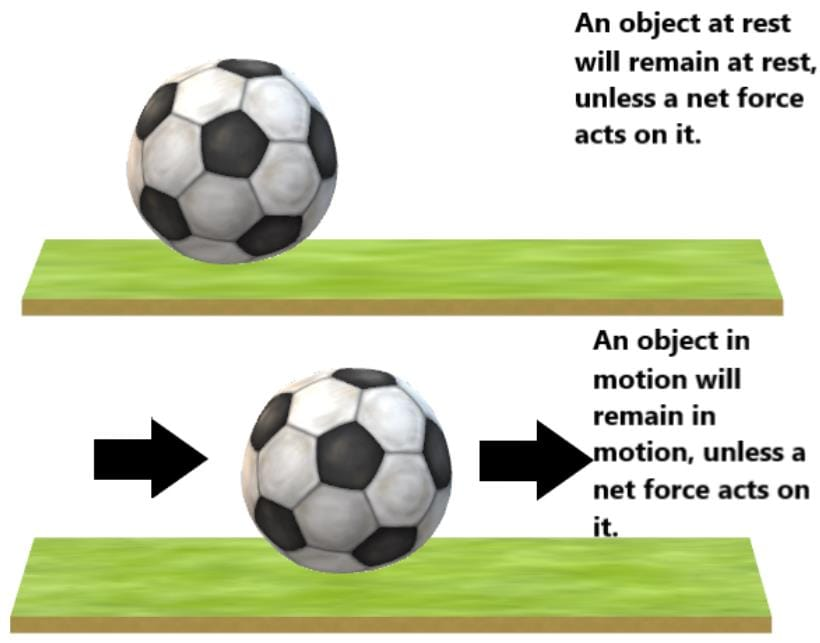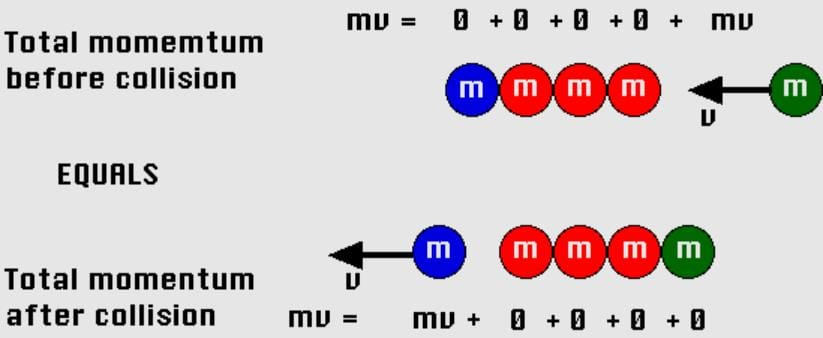JEE  >  Test: Laws of Motion - 1

# Test: Laws of Motion - 1

Test Description

## 30 Questions MCQ Test Physics Class 11 | Test: Laws of Motion - 1

Test: Laws of Motion - 1 for JEE 2023 is part of Physics Class 11 preparation. The Test: Laws of Motion - 1 questions and answers have been prepared according to the JEE exam syllabus.The Test: Laws of Motion - 1 MCQs are made for JEE 2023 Exam. Find important definitions, questions, notes, meanings, examples, exercises, MCQs and online tests for Test: Laws of Motion - 1 below.
Solutions of Test: Laws of Motion - 1 questions in English are available as part of our Physics Class 11 for JEE & Test: Laws of Motion - 1 solutions in Hindi for Physics Class 11 course. Download more important topics, notes, lectures and mock test series for JEE Exam by signing up for free. Attempt Test: Laws of Motion - 1 | 30 questions in 30 minutes | Mock test for JEE preparation | Free important questions MCQ to study Physics Class 11 for JEE Exam | Download free PDF with solutions
 1 Crore+ students have signed up on EduRev. Have you?
Test: Laws of Motion - 1 - Question 1

### A stone of mass 0.25 kg tied to the end of a string is whirled round in a circle of radius 1.5 m with a speed of 40 rev./min in a horizontal plane. The speed is then increased beyond the maximum permissible value, and the string breaks suddenly, which of the following correctly describes the trajectory of the stone after the string breaks.

Detailed Solution for Test: Laws of Motion - 1 - Question 1
• The stone is moving in a circular path with constant speed, this is uniform circular motion.
• In uniform circular motion, at any instant velocity (magnitude speed) acts tangentially to the circular path.
• Hence, when string is cut the stone will fly off tangentially to circular path with velocity of magnitude speed.Test: Laws of Motion - 1 - Question 2

### A man of mass 70 kg stands on a weighing scale in a lift which is moving upwards with a uniform speed of 10 m s−1, what would be the reading on the scale?

Detailed Solution for Test: Laws of Motion - 1 - Question 2

Mass of the man, m = 70 kg
Acceleration, a = 0
Using Newton’s second law of motion, We can write the equation of motion as,
R – mg = ma
∴ R = mg = 70 × 10 = 700 N
∴ the weighing scale = 700 / g = 700 / 10 = 70 kg

Test: Laws of Motion - 1 - Question 3

### Give the magnitude and direction of the net force acting on a stone of mass 0.1 kg lying on the floor of a train which is accelerating with 1 ms−2, the stone being at rest relative to the train. Neglect air resistance.

Detailed Solution for Test: Laws of Motion - 1 - Question 3

As the stone is lying on the floor of the train, its acceleration is same as that of the train.
∴ Force acting on stone,
F = ma = 0.1 x 1 = 0.1 N.

• This force is along the horizontal direction of motion of the train.
• Note that in each case, the weight of the stone is being balanced by the normal reaction.
• Pseudo force is considered when we are not in the same frame as that of the object.
Test: Laws of Motion - 1 - Question 4

Newton’s third law states that when two bodies interact.

Detailed Solution for Test: Laws of Motion - 1 - Question 4
• The third law states that all forces between two objects exist in equal magnitude and opposite direction.
• If one object A exerts a force FA on a second object B, then B simultaneously exerts a force FB on A, and the two forces are equal in magnitude and opposite in direction, FA = −FB
• Newton's third Law:Test: Laws of Motion - 1 - Question 5

Force is required

Detailed Solution for Test: Laws of Motion - 1 - Question 5
• Force is required to start a stationary object and to stop a moving object due to inertia.
• Inertia is a property of matter by which it continues in its existing state of rest or uniform motion in a straight line, unless that state is changed by an external force.
• This is also called law of inertia or newton's first law of motion.
• Newton's first Law:Test: Laws of Motion - 1 - Question 6

According to Newton's third law of motion, the action and reaction forces are

Detailed Solution for Test: Laws of Motion - 1 - Question 6
• When one body exerts a force on a second body, the second body simultaneously exerts a force equal in magnitude and opposite in direction on the first body.
• To every action there is always opposed an equal reaction or the mutual actions of two bodies upon each other are always equal and opposite in direction.
Test: Laws of Motion - 1 - Question 7

According to first law of motion

Detailed Solution for Test: Laws of Motion - 1 - Question 7

If net force act on a body then acceleration of the body will also be zero. Hence velocity will not be changed i.e. it continues in its existing state of rest or uniform motion in a straight line.

Test: Laws of Motion - 1 - Question 8

Give the magnitude and direction of the net force acting on a stone of mass 0.1 kg lying on the floor of a train which is accelerating with 1 ms−2, the stone being at rest relative to the train. Neglect air resistance.

Detailed Solution for Test: Laws of Motion - 1 - Question 8

Weight of the stone is balanced by the reaction of the floor. The only acceleration is provided by the horizontal motion of the train.

a = 1ms-2

Force in horizontal direction

F = ma = 0.1 x 1 = 0.1N

Test: Laws of Motion - 1 - Question 9

A man of mass 70 kg stands on a weighing scale in a lift which is moving upwards with a uniform acceleration of 10 ms−2 what would be the reading on the scale?

Detailed Solution for Test: Laws of Motion - 1 - Question 9

According to Newton's second Law of motion,
Apparent weight, R = m(a+g)

= 70(10+10)

= 1400N

mass = R/g

= 1400/10

= 140Kg

Test: Laws of Motion - 1 - Question 10

A helicopter of mass 1000 kg rises with a vertical acceleration of 15 ms−2. The crew and the passengers weigh 300 kg. Give the magnitude and direction of the force on the floor by the crew and passengers,

Detailed Solution for Test: Laws of Motion - 1 - Question 10
• The moving helicopter is a non-inertial frame and hence we do need to apply pseudo force onto the body so as to solve equations with Newton's laws.
• The pseudo force needed to be applied, f = ma = 300 x 15 N = 4500N
• Thus the net downward force due to the passengers is 7500N.
Test: Laws of Motion - 1 - Question 11

A train runs along an unbanked circular track of radius 30 m at a speed of 54 km/h. The mass of the train is 106 kg. What is the angle of banking required to prevent wearing out of the rail?

Detailed Solution for Test: Laws of Motion - 1 - Question 11

Radius of the circular track, r = 30 m

Speed of the train, v = 54 km/h = 15 m/s

Mass of the train, m = 106 kg

The centripetal force is provided by the lateral thrust of the rail on the wheel.
As per Newton’s third law of motion, the wheel exerts an equal and opposite force on the rail.

The angle of banking θ, is related to the radius (r) and speed (v) by the relation:

tan θ = v2 / rg

= 152 / (30 x 10)

= 225 / 300

⇒ θ = tan-1 (0.75) = 36.870

Therefore, the angle of banking is about 36.87degree = 37degree.

Test: Laws of Motion - 1 - Question 12

According to second law of motion

Detailed Solution for Test: Laws of Motion - 1 - Question 12
• According to Newton s Second Law of Motion, also known as the Law of Force and Acceleration, a force upon an object causes it to accelerate according to the formula net force = mass x acceleration.
• It states that the force applied on a body is proportional to the rate of change of momentum of the body.
• So the acceleration of the object is directly proportional to the force and inversely proportional to the mass.
Test: Laws of Motion - 1 - Question 13

According to the law of conservation of momentum,

Detailed Solution for Test: Laws of Motion - 1 - Question 13

When no external forces act on a system of several interacting particles, then the total momentum of system is conserved.
Law of conservation of Momentum:Test: Laws of Motion - 1 - Question 14

A constant retarding force of 50 N is applied to a body of mass 20 kg moving initially with a speed of 15 m s−1How long does the body take to stop?

Detailed Solution for Test: Laws of Motion - 1 - Question 14

According to Newton's second Law
Force = mass x accleration
F = m.a
= m.v/t
t  = m.v/F
= 20x15/50
= 6 sec

Test: Laws of Motion - 1 - Question 15

A man of mass 70 kg stands on a weighing scale in a lift which is moving upwards with a uniform acceleration of 5 ms−2 what would be the reading on the scale?

Detailed Solution for Test: Laws of Motion - 1 - Question 15

Mass of the man, m = 70 kg

Acceleration, a = 5 m/s2 upward

Using Newton’s second law of motion, we can write the equation of motion as:

R – mg = ma

R = m(g + a)

= 70 (10 + 5) = 70 x 15

= 1050 N

∴ Reading on the weighing scale = 1050 / g = 1050 / 10 = 105 kg

Test: Laws of Motion - 1 - Question 16

A monkey of mass 40 kg climbs on a rope which can stand a maximum tension of 600 N. What is the tension in the rope if the monkey climbs up with an acceleration of 6 ms−2

Detailed Solution for Test: Laws of Motion - 1 - Question 16

Mass of the monkey, m = 40 kg
Acceleration due to gravity, g = 10 m/s
Maximum tension that the rope can bear, Tmax = 600 N
Acceleration of the monkey, a = 6 m/s2 upward
Using Newtons second law of motion, we can write the equation of motion as:
T  mg = ma
T = m(g + a)
= 40 (10 + 6)
= 640 N

Test: Laws of Motion - 1 - Question 17

Force is a

Detailed Solution for Test: Laws of Motion - 1 - Question 17

Newton's second law of motion gives the quantitative definition of force. The acceleration of an object as produced by a net force is directly proportional to the magnitude of the net force, in the same direction as the net force, and inversely proportional to the mass of the object.

Test: Laws of Motion - 1 - Question 18

When bodies are in contact with each other then

Detailed Solution for Test: Laws of Motion - 1 - Question 18
• According to newton's third law of motion for every action there is always equal and opposite reaction or the mutual actions of two bodies upon each other.
• Whenever two objects interact with each other, then the pair of forces acting on them are equal in magnitude and opposite in direction. Contact forces are mutual forces (action and reaction).
Test: Laws of Motion - 1 - Question 19

A rocket with a lift-off mass 20,000 kg is blasted upwards with an initial acceleration of 5.0 ms-2. Calculate the initial thrust (force) of the blast.

Detailed Solution for Test: Laws of Motion - 1 - Question 19

Initial thrust = upthrust required to impart acceleration + uthrust to overcome gravity

⇒ ma + mg = m(a+g)
⇒ 20000(5+10) = 300000 N

Test: Laws of Motion - 1 - Question 20

Two bodies of masses 10 kg and 20 kg respectively kept on a smooth, horizontal surface are tied to the ends of a light string. a horizontal force F = 600 N is applied to the 20 kg mass so as to pull it. What is the tension in the string?

Detailed Solution for Test: Laws of Motion - 1 - Question 20

For some instance assume both masses as one system, thus we get that
600 = 30a
Where a is the common acceleration of the system.
Now if we consider the 10kg block we get that
T = 10a
And a = 20m/s
Thus we get T = 200N

Test: Laws of Motion - 1 - Question 21

A monkey of mass 40 kg climbs on a rope which can stand a maximum tension of 600 N. What is the tension in the rope if the monkey climbs down with an acceleration of 4 ms−2

Detailed Solution for Test: Laws of Motion - 1 - Question 21

Mass of the monkey, m = 40 kg
Acceleration due to gravity, g = 10 m/s
Maximum tension that the rope can bear, Tmax = 600 N
Acceleration of the monkey, a = 4 m/s2 downward
Using Newton’s second law of motion, we can write the equation of motion as:
mg T = ma
T = m (g- a)
= 40(10-4)
= 240 N

Test: Laws of Motion - 1 - Question 22

When several forces act on a body

Detailed Solution for Test: Laws of Motion - 1 - Question 22

When an object has several forces acting on it, the effect of force is the same as one force acting on the object in a certain direction and this overall force is called the ‘resultant force’. The resultant force is essential to change the velocity of an object.

Test: Laws of Motion - 1 - Question 23

Friction is

Detailed Solution for Test: Laws of Motion - 1 - Question 23
• Friction opposes the relative motion between the surfaces in contact with each other. Friction is the force that opposes relative motion or tendency of relative motion between two surfaces in contact.
• When two surfaces move relative to each other or they have a tendency to move relative to each other, at the point (or surface) of contact, there appears a force which opposes this relative motion or tendency of relative motion between two surfaces in contact.
• It acts on both the surfaces in contact with equal magnitude and opposite directions (Newton's 3rd law). also Friction force tries to stop relative motion between two surfaces in contact.
Test: Laws of Motion - 1 - Question 24

A truck starts from rest and accelerates uniformly at 2.0 ms−2. At t = 10 s, a stone is dropped by a person standing on the top of the truck (6 m high from the ground). What is the magnitude of acceleration (in ms−2 ) of the stone at t = 11s? (Neglect air resistance.)

Detailed Solution for Test: Laws of Motion - 1 - Question 24

Initial velocity of the truck, u = 0

Acceleration, a = 2 m/s2

Time, t = 10 s

As per the first equation of motion, final velocity is given as:

v = u + at

⇒ 0 + 2 X 10 = 20 m/s

The final velocity of the truck and hence, of the stone is 20 m/s.

At t = 11 s, the horizontal component (vx) of velocity, in the absence of air resistance, remains unchanged, i.e.,

vx = 20 m/s

The vertical component (vy) of velocity of the stone is given by the first equation of motion as:

vy = u + ayδt

Where, δt = 11 – 10 = 1 s and ay = g = 10 m/s2

∴vy = 0 + 10 X 1 = 10 m/s

Test: Laws of Motion - 1 - Question 25

A monkey of mass 40 kg climbs on a rope which can stand a maximum tension of 600 N. What is the tension in the rope if the monkey climbs up with a uniform speed of 5 ms−1

Detailed Solution for Test: Laws of Motion - 1 - Question 25

When monkey climbs with uniform speed, the acceleration is zero, so

T = mg = 40x10 = 400 N

Test: Laws of Motion - 1 - Question 26

A stationary ball weighing 0.25kg acquires a speed of 10m/s when hit by a hockey stick. The impulse imparted to the ball is

Detailed Solution for Test: Laws of Motion - 1 - Question 26

Initial momentum of the ball,
Pi​ = 0
Final momentum of the ball,
Pf  = mv
⇒ 0.25×10 = 2.5 kg m/s
Impulse imparted on the ball,
I = Pf − Pi
⇒ I = 2.5 − 0 = 2.5 N s

Test: Laws of Motion - 1 - Question 27

Static friction force

Detailed Solution for Test: Laws of Motion - 1 - Question 27
• It is the maximum friction, where a body just starts to move over the surface.
• For example a large block of mass m is placed on a horizontal table. Apply a small force the block does not move due to the friction force which balances the applied force.
• Now Gradually increase the applied force until it start moving. This maximum applied force acting in opposite direction is called as Static Friction
Test: Laws of Motion - 1 - Question 28

A bob of mass 0.1 kg hung from the ceiling of a room by a string 2 m long is set into oscillation. The speed of the bob at its mean position is 1 ms−1. What is the trajectory of the bob if the string is cut when the bob is at one of its extreme positions

Detailed Solution for Test: Laws of Motion - 1 - Question 28

At the extreme position of the oscillation, the speed of the bob is zero. So the bob is momentarily at rest. if the string is cut, the bob will fall vertically downwards.

Test: Laws of Motion - 1 - Question 29

If second law is applied to a rigid body

Detailed Solution for Test: Laws of Motion - 1 - Question 29

The net external force on the rigid body is always equal to the total mass times the translational acceleration (i.e., Newton's second law holds for the translational motion, even when the net external torque is nonzero, and/or the body rotates).

Test: Laws of Motion - 1 - Question 30

Rolling friction is

Detailed Solution for Test: Laws of Motion - 1 - Question 30

Rolling friction is always less than static friction because in order for an object to roll the force of friction between it and surface must be large enough to keep the object from sliding. Hence rolling friction is always greater than static force.

## Physics Class 11

127 videos|464 docs|210 tests
 Use Code STAYHOME200 and get INR 200 additional OFF Use Coupon Code
Information about Test: Laws of Motion - 1 Page
In this test you can find the Exam questions for Test: Laws of Motion - 1 solved & explained in the simplest way possible. Besides giving Questions and answers for Test: Laws of Motion - 1, EduRev gives you an ample number of Online tests for practice

## Physics Class 11

127 videos|464 docs|210 tests